# 计算机视觉之损失函数

Posted by jjx on February 27, 2017

#### 多类支持向量机损失 Multiclass Support Vector Machine Loss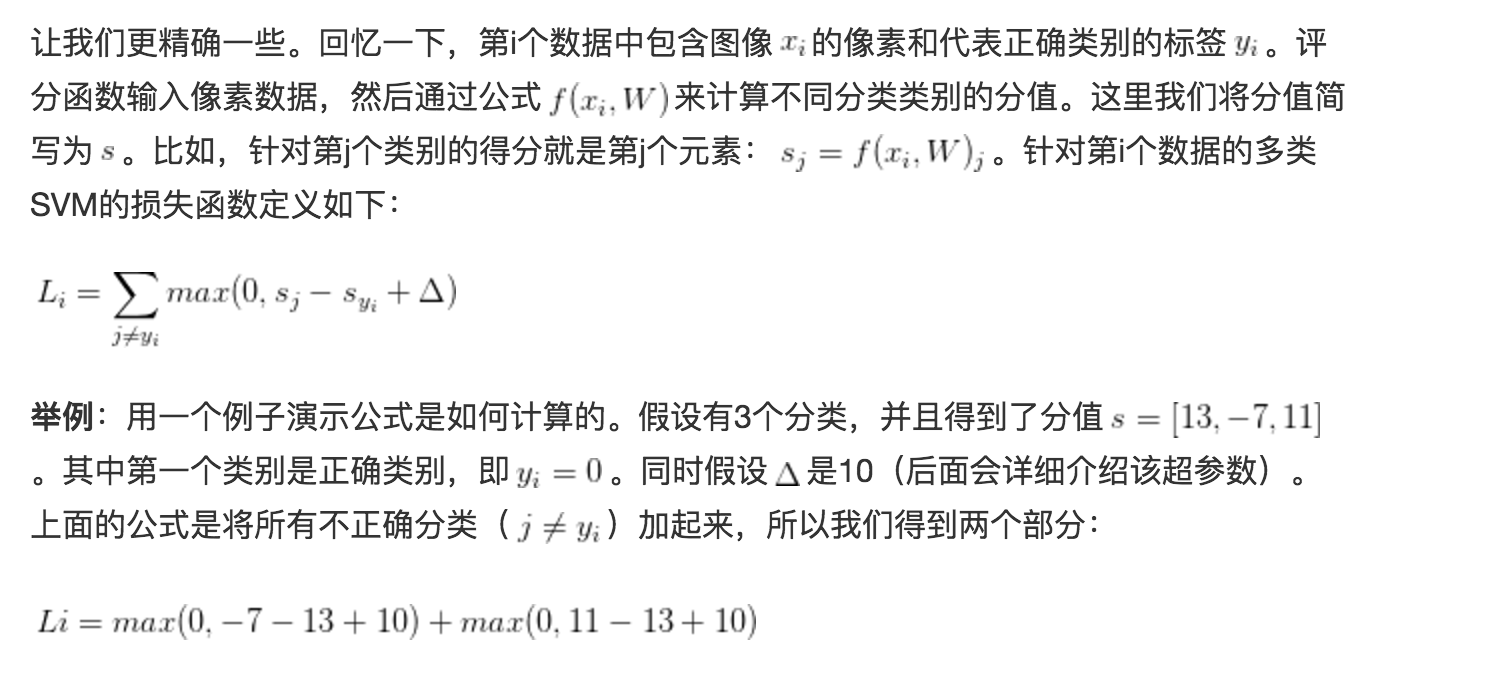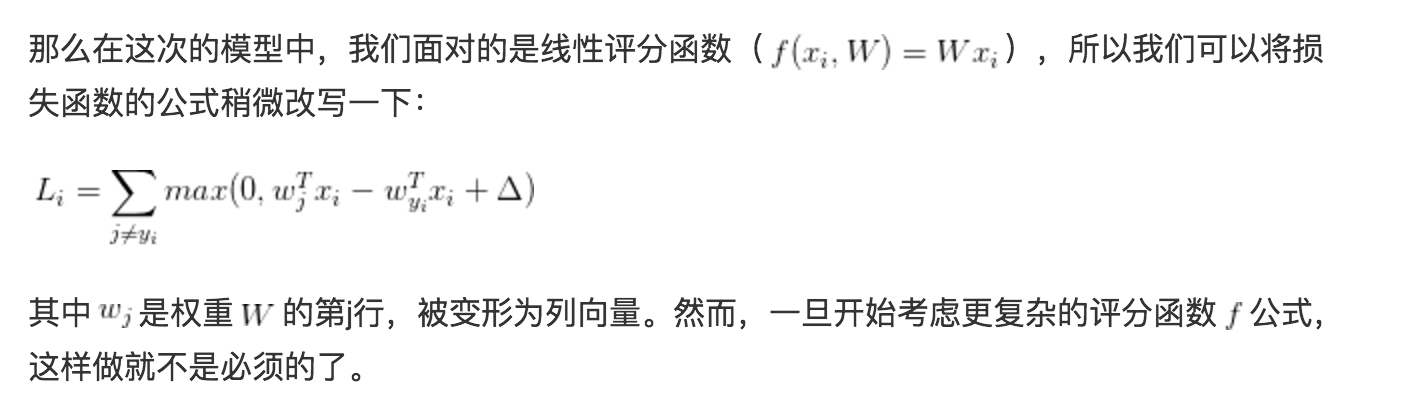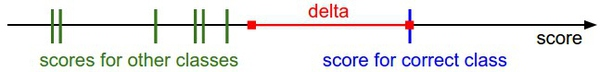$R(W)=\sum_k\sum_l{W_{k,l}^2}$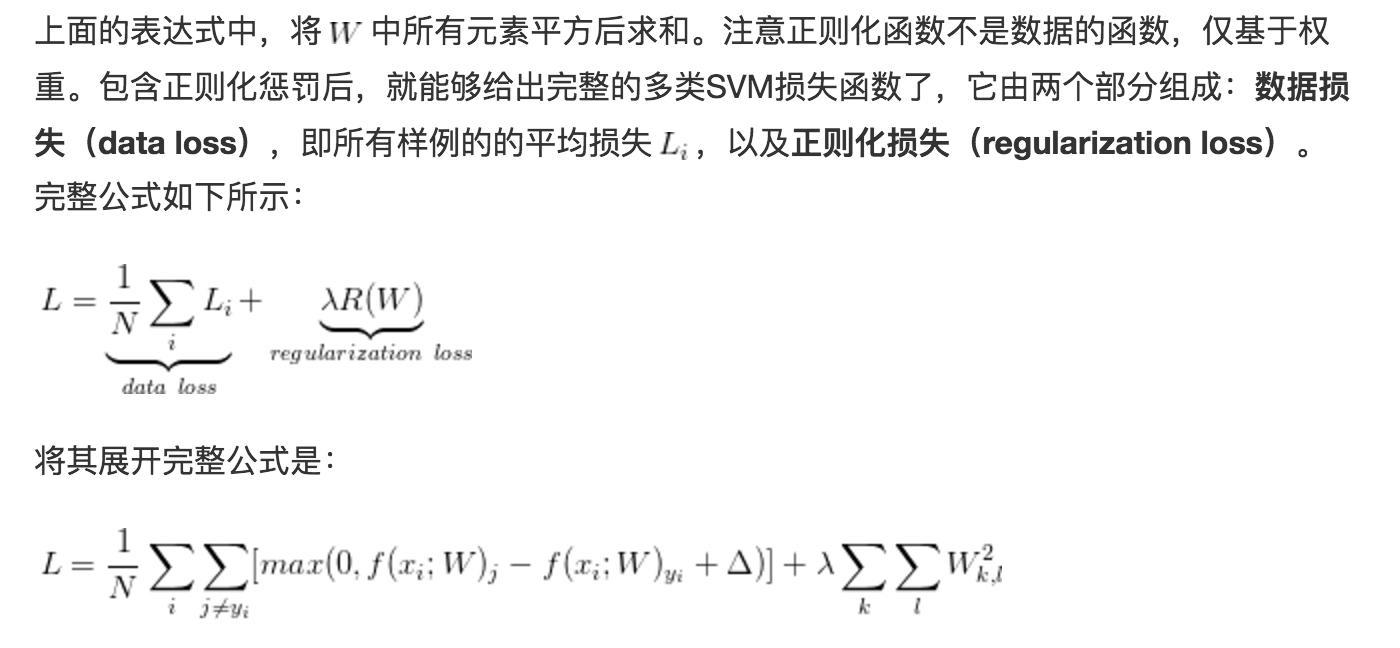def L_i(x, y, W):
"""
unvectorized version. Compute the multiclass svm loss for a single example (x,y)
- x is a column vector representing an image (e.g. 3073 x 1 in CIFAR-10)
with an appended bias dimension in the 3073-rd position (i.e. bias trick)
- y is an integer giving index of correct class (e.g. between 0 and 9 in CIFAR-10)
- W is the weight matrix (e.g. 10 x 3073 in CIFAR-10)
"""
delta = 1.0 # see notes about delta later in this section
scores = W.dot(x) # scores becomes of size 10 x 1, the scores for each class
correct_class_score = scores[y]
D = W.shape # number of classes, e.g. 10
loss_i = 0.0
for j in xrange(D): # iterate over all wrong classes
if j == y:
# skip for the true class to only loop over incorrect classes
continue
# accumulate loss for the i-th example
loss_i += max(0, scores[j] - correct_class_score + delta)
return loss_i

def L_i_vectorized(x, y, W):
"""
A faster half-vectorized implementation. half-vectorized
refers to the fact that for a single example the implementation contains
no for loops, but there is still one loop over the examples (outside this function)
"""
delta = 1.0
scores = W.dot(x)
# compute the margins for all classes in one vector operation
margins = np.maximum(0, scores - scores[y] + delta)
# on y-th position scores[y] - scores[y] canceled and gave delta. We want
# to ignore the y-th position and only consider margin on max wrong class
margins[y] = 0
loss_i = np.sum(margins)
return loss_i

def L(X, y, W):
"""
fully-vectorized implementation :
- X holds all the training examples as columns (e.g. 3073 x 50,000 in CIFAR-10)
- y is array of integers specifying correct class (e.g. 50,000-D array)
- W are weights (e.g. 10 x 3073)
"""
# evaluate loss over all examples in X without using any for loops
# left as exercise to reader in the assignment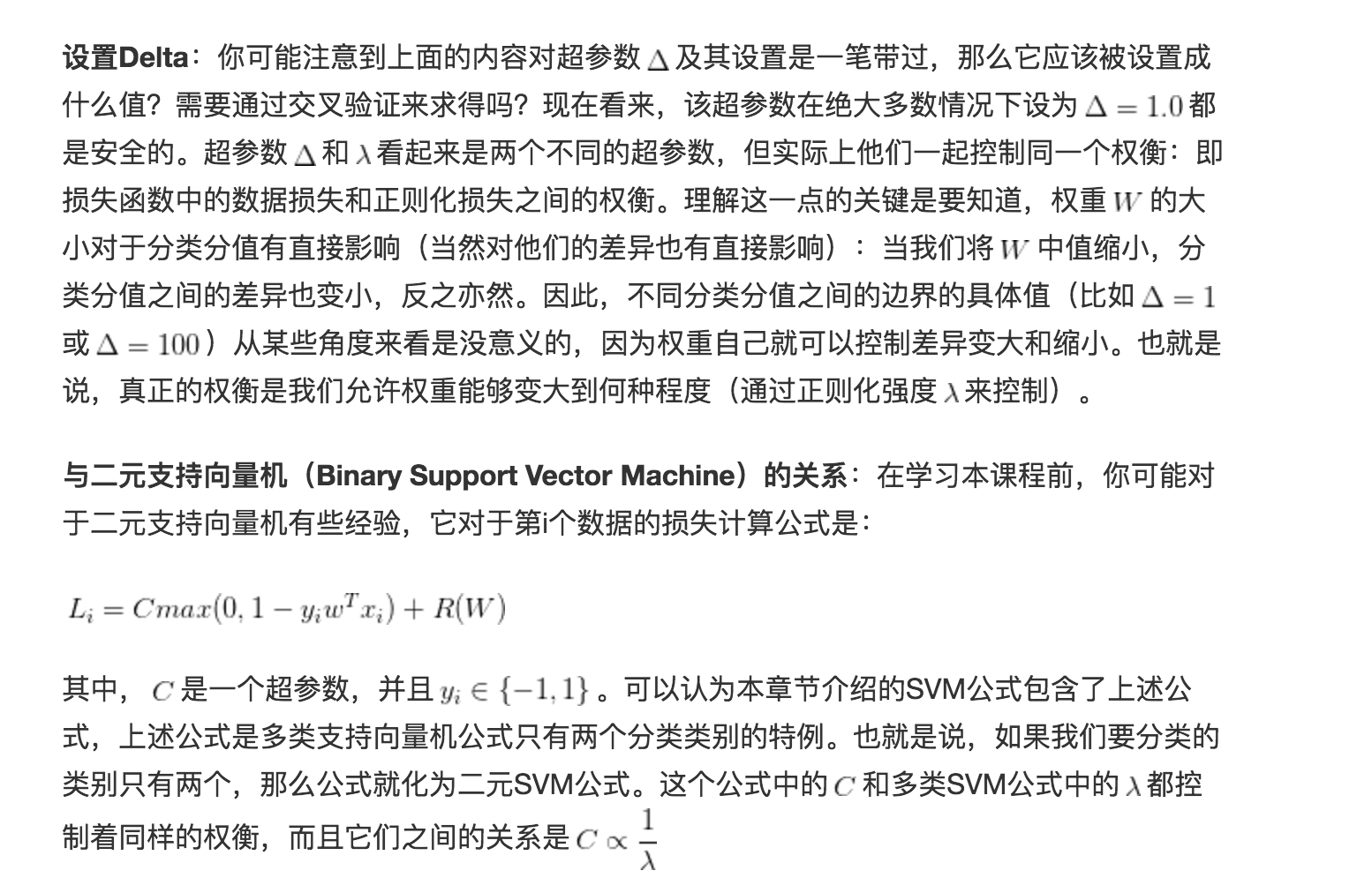#### Softmax分类器

SVM是最常用的两个分类器之一，而另一个就是Softmax分类器，它的损失函数与SVM的损失函数不同。对于学习过二元逻辑回归分类器的读者来说，Softmax分类器就可以理解为逻辑回归分类器面对多个分类的一般化归纳。SVM将输出$f(x_i,W)$作为每个分类的评分（因为无定标，所以难以直接解释）。与SVM不同，Softmax的输出（归一化的分类概率）更加直观，并且从概率上可以解释，这一点后文会讨论。在Softmax分类器中，函数$f(x_i;W)=Wx_i$映射保持不变，但将这些评分值视为每个分类的未归一化的对数概率，并且将折叶损失（hinge loss）替换为交叉熵损失（cross-entropy loss）。公式如下：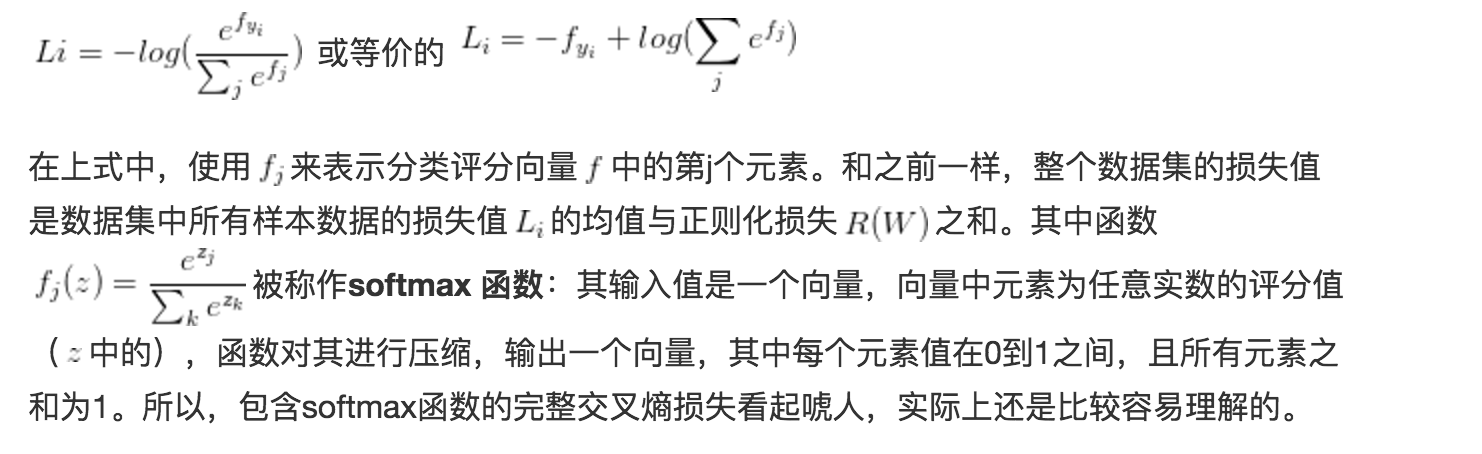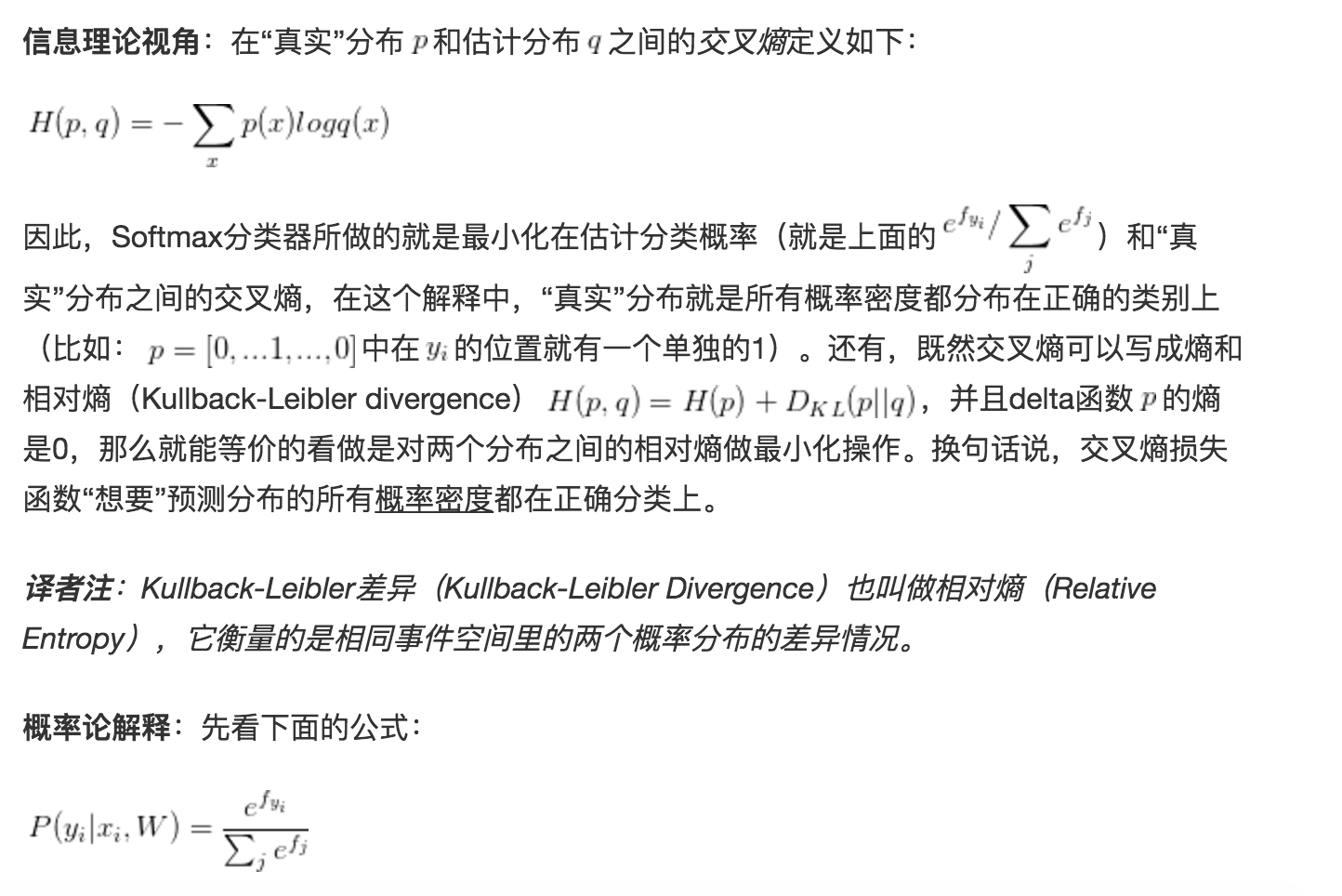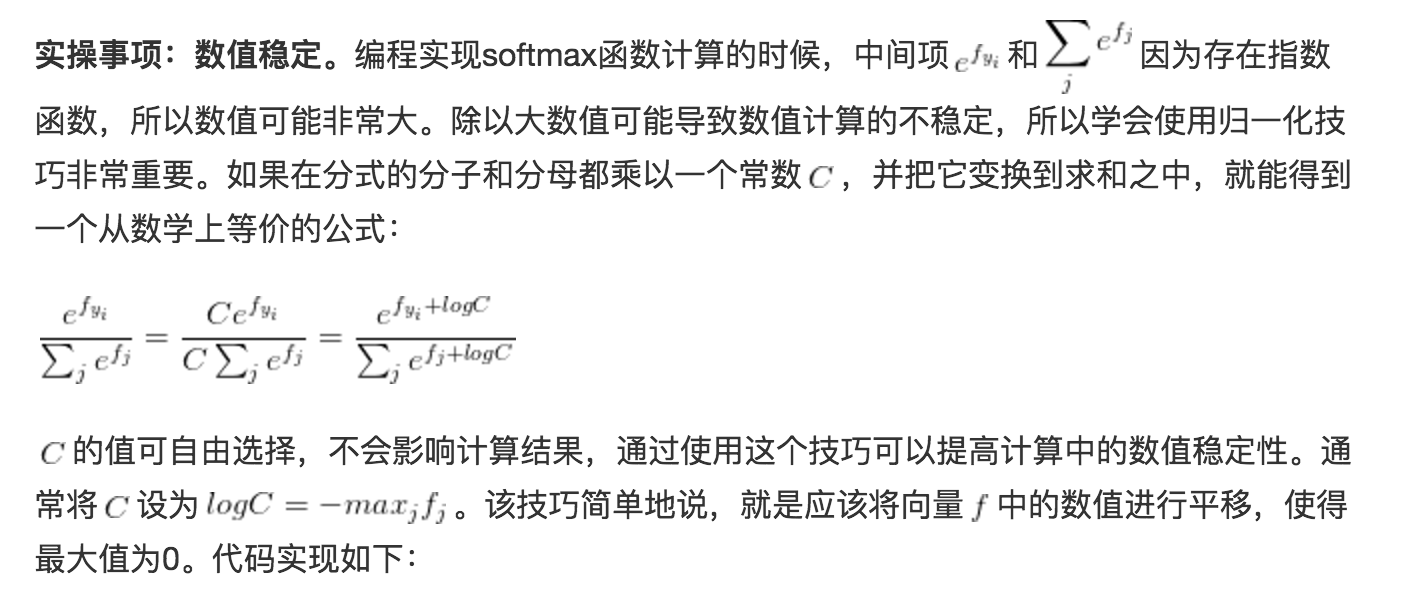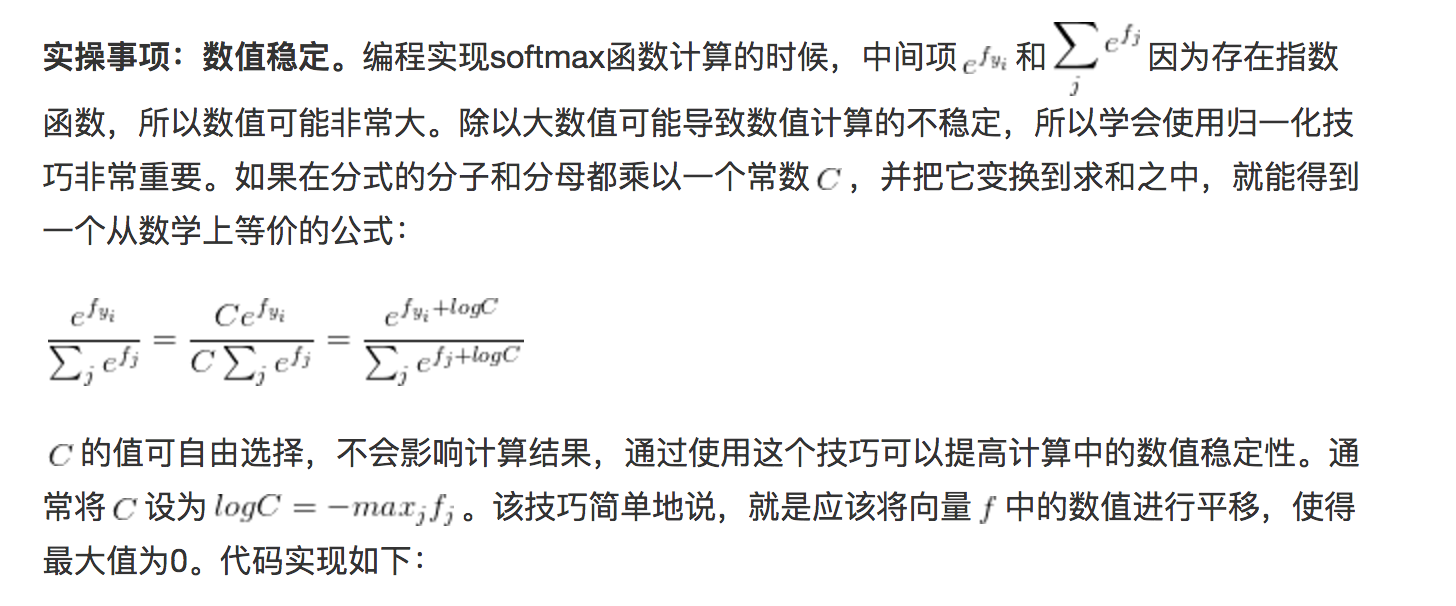f = np.array([123, 456, 789]) # 例子中有3个分类，每个评分的数值都很大
p = np.exp(f) / np.sum(np.exp(f)) # 不妙：数值问题，可能导致数值爆炸

# 那么将f中的值平移到最大值为0：
f -= np.max(f) # f becomes [-666, -333, 0]
p = np.exp(f) / np.sum(np.exp(f)) # 现在OK了，将给出正确结果


#### SVM和Softmax的比较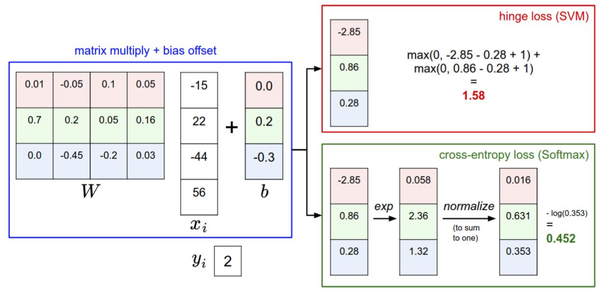• SVM下，我们能完成类别的判定，但是实际上我们得到的类别得分，大小顺序表示着所属类别的排序，但是得分的绝对值大小并没有特别明显的物理含义。
• Softmax分类器中，结果的绝对值大小表征属于该类别的概率。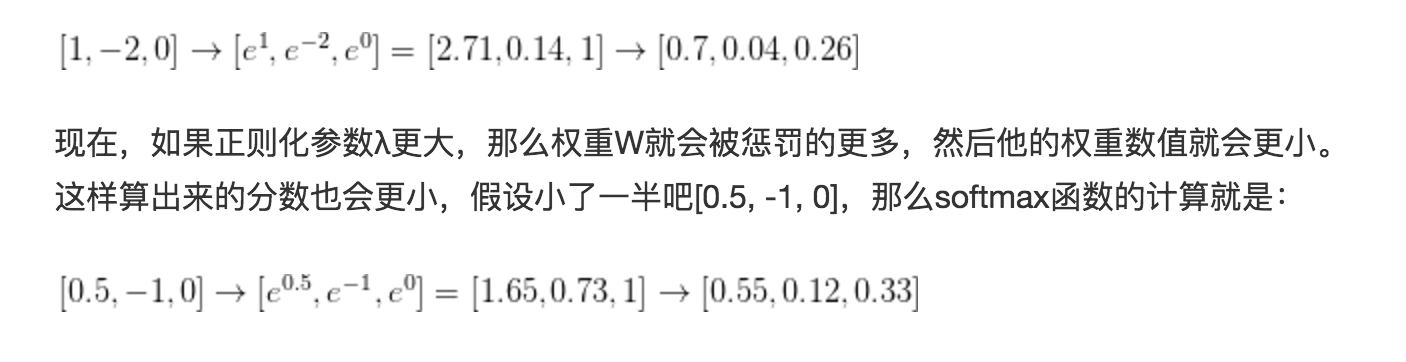SVM其实并不在乎每个类别得到的绝对得分大小，举个例子说，我们现在对三个类别，算得的得分是[10, -2, 3]，实际第一类是正确结果，而设定Δ=1，那么10-3=7已经比1要大很多了，那对SVM而言，它觉得这已经是一个很标准的答案了，完全满足要求了，不需要再做其他事情了，结果是 [10, -100, -100] 或者 [10, 9, 9]，它都是满意的。